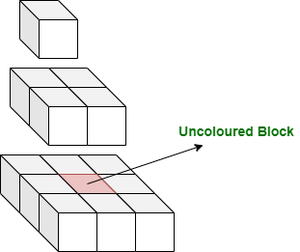Open In App

# Count of uncolored cells in a Pyramid of length H formed using cubes

Given a pyramid, formed using unit area cubes, of given height H. The pyramid is then placed on the ground and painted from outside. The task is to find the count of cubes that are left uncoloured.

Examples:

Input: H = 3
Output: 1
Explanation:Input: H = 1
Output: 0

Naive Approach: The number of cubes that will remain uncoloured is the number of cubes that are not present in the boundary of the pyramid. So, for every layer of cubes at height h, the number of uncoloured cubes iswhere h>1. So, add the number of uncoloured cubes at every level to get the answer.

Below is the implementation of the above approach:

## C++

 `// C++ program for the above problem` `#include ``using` `namespace` `std;` `// Function to return the number of``// uncoloured cubes``int` `uncolouredCubes(``int` `H)``{` `    ``// To store the number of uncoloured``    ``// cubes``    ``int` `res = 0;` `    ``for` `(``int` `h = 2; h <= H; h++) {``        ``res += (h - 2) * (h - 2);``    ``}` `    ``return` `res;``}` `// Driver Code``int` `main()``{``    ``int` `H = 3;``    ``cout << uncolouredCubes(H);``}`

## Java

 `// Java program for the above problem`  `class` `GFG{` `  ``// Function to return the number of``  ``// uncoloured cubes``  ``static` `int` `uncolouredCubes(``int` `H)``  ``{` `      ``// To store the number of uncoloured``      ``// cubes``      ``int` `res = ``0``;` `      ``for` `(``int` `h = ``2``; h <= H; h++) {``          ``res += (h - ``2``) * (h - ``2``);``      ``}` `      ``return` `res;``  ``}` `  ``// Driver Code``  ``public` `static` `void` `main(String [] args)``  ``{``      ``int` `H = ``3``;``      ``System.out.println(uncolouredCubes(H));``  ``}``  ` `}` `// This code is contributed by ihritik`

## Python3

 `# python program for the above problem` `# Function to return the number of``# uncoloured cubes``def` `uncolouredCubes(H):`  `    ``# To store the number of uncoloured``    ``# cubes``    ``res ``=` `0` `    ``for` `h ``in` `range``(``2``, H ``+` `1``):``        ``res ``=` `res ``+` `(h ``-` `2``) ``*` `(h ``-` `2``)``    `  `    ``return` `res`  `# Driver Code` `H ``=` `3``print``(uncolouredCubes(H))` `# This code is contributed by ihritik`

## C#

 `// C# program for the above problem` `using` `System;``class` `GFG{` `  ``// Function to return the number of``  ``// uncoloured cubes``  ``static` `int` `uncolouredCubes(``int` `H)``  ``{` `      ``// To store the number of uncoloured``      ``// cubes``      ``int` `res = 0;` `      ``for` `(``int` `h = 2; h <= H; h++) {``          ``res += (h - 2) * (h - 2);``      ``}` `      ``return` `res;``  ``}` `  ``// Driver Code``  ``public` `static` `void` `Main()``  ``{``      ``int` `H = 3;``      ``Console.WriteLine(uncolouredCubes(H));``  ``}``  ` `}` `// This code is contributed by ihritik`

## Javascript

 ``

Output

`1`

Time Complexity: O(N)
Auxiliary Space: O(1)

Efficient Approach: As the number of uncoloured cubes in each layer at height h is, so this problem can be reduced to find the sum of the squares of the first (H-2) natural numbers. So, the answer is (n * (n + 1) * (2n + 1)) / 6 where n=H-2.

## C++

 `// C++ program for the above problem` `#include ``using` `namespace` `std;` `// Function to return the number of``// uncoloured cubes``int` `uncolouredCubes(``int` `H)``{` `    ``// If H is less than 2, then``    ``// returning 0``    ``if` `(H < 2) {``        ``return` `0;``    ``}` `    ``int` `n = H - 2;` `    ``// Sum of the squares of first``    ``// n natural numbers``    ``return` `(n * (n + 1) * (2 * n + 1)) / 6;``}` `// Driver Code``int` `main()``{``    ``int` `H = 3;``    ``cout << uncolouredCubes(H);``}`

## Java

 `// Java program for the above problem``import` `java.util.*;``public` `class` `GFG``{``  ` `// Function to return the number of``// uncoloured cubes``static` `int` `uncolouredCubes(``int` `H)``{``  ` `    ``// If H is less than 2, then``    ``// returning 0``    ``if` `(H < ``2``) {``        ``return` `0``;``    ``}``    ``int` `n = H - ``2``;` `    ``// Sum of the squares of first``    ``// n natural numbers``    ``return` `(n * (n + ``1``) * (``2` `* n + ``1``)) / ``6``;``}` `// Driver Code``public` `static` `void` `main(String args[])``{``    ``int` `H = ``3``;``    ``System.out.println(uncolouredCubes(H));``}``}` `// This code is contributed by Samim Hossain Mondal`

## Python3

 `# Python program for the above problem` `# Function to return the number of``# uncoloured cubes``def` `uncolouredCubes(H):` `  ``# If H is less than 2, then``  ``# returning 0``    ``if` `(H < ``2``):``        ``return` `0``;` `    ``n ``=` `H ``-` `2``;` `    ``# Sum of the squares of first``    ``# n natural numbers``    ``return` `(n ``*` `(n ``+` `1``) ``*` `(``2` `*` `n ``+` `1``)) ``/` `6``;` `# Driver Code``H ``=` `3``;``print``((``int``)(uncolouredCubes(H)));` `# This code is contributed by Saurabh Jaiswal.`

## C#

 `// C# program for the above problem``using` `System;``class` `GFG``{``// Function to return the number of``// uncoloured cubes``static` `int` `uncolouredCubes(``int` `H)``{``    ``// If H is less than 2, then``    ``// returning 0``    ``if` `(H < 2) {``        ``return` `0;``    ``}``    ``int` `n = H - 2;` `    ``// Sum of the squares of first``    ``// n natural numbers``    ``return` `(n * (n + 1) * (2 * n + 1)) / 6;``}` `// Driver Code``public` `static` `void` `Main()``{``    ``int` `H = 3;``    ``Console.Write(uncolouredCubes(H));``}``}``// This code is contributed by Samim Hossain Mondal`

## Javascript

 `// Javascript program for the above problem` `// Function to return the number of``// uncoloured cubes``function` `uncolouredCubes(H)``{` `  ``// If H is less than 2, then``  ``// returning 0``  ``if` `(H < 2) {``    ``return` `0;``  ``}` `  ``let n = H - 2;` `  ``// Sum of the squares of first``  ``// n natural numbers``  ``return` `(n * (n + 1) * (2 * n + 1)) / 6;``}` `// Driver Code` `let H = 3;``document.write(uncolouredCubes(H));` `// This code is contributed by gfgking.`

Output

`1`

Time Complexity: O(1)
Auxiliary Space: O(1)